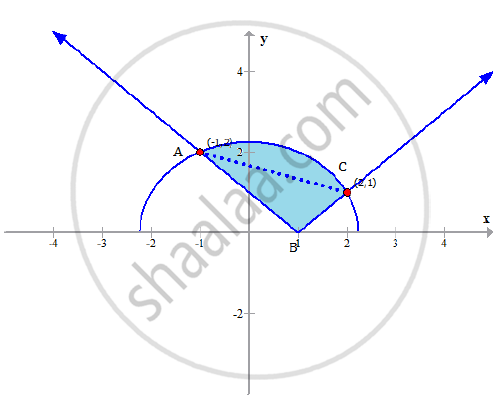# Sketch the region bounded by the curves y=√(5-x^2) and y=|x-1| and find its area using integration. - Mathematics

Sketch the region bounded by the curves y=sqrt(5-x^2) and y=|x-1| and find its area using integration.

#### Solution

Consider the given equation

y=sqrt(5-x^2)

This equation represents a semicircle with centre at

the origin and radius = sqrt5 units

Given that the region is bounded by the above
semicircle and the line y = |x-1|
Let us find the point of intersection of the
given curve meets the line y= |x - 1|

=>sqrt(5-x^2)=|x-1|

Squaring both the sides, we have,

5-x^2=|x-1|^2

=>5-x^2=x^2+1-2x

=>2x^2-2x-5+1=0

=>2x^2-2x-4=0

=>x^2-x-2=0

=>x^2-2x+x-2=0

=>x(x-2)+1(x-2)=0

=>(x+1)(x-2)=0

=>x=-1, x=2

When x = -1,y = 2
When x = 2,y = 1
Consider the following figure.

Thus the intersection points are ( -1,2) and (2,1)
Consider the following sketch of the bounded region.Required Area, A= int_(-1)^2(y_2-y_1)dx

==int_(-1)^1[sqrt(5-x^2)+(x-1)]dx+int_1^2[sqrt(5-x^2)-(x-1)]dx

=int_(-1)^1sqrt(5-x^2)dx+int_(-1)^1xdx-int_(-1)^1dx+int_1^2sqrt(5-x^2)dx-int_1^2xdx+int_1^2dx

=[x/2sqrt(5-x^2)+5/2sin^-1(x/sqrt5)]_(-1)^1+(x^2/2)_(-1)^1-(x)_(-1)^1+[x/2sqrt(5-x^2)+5/2sin^-1(x/sqrt5)]_1^2-(x^2/2)_(1)^2+(x)_(1)^2

=5/2 sin^-1 (1/sqrt5)+5/2 sin^-1 (2/sqrt5)-1/2

Required area= [5/2 sin^-1 (1/sqrt5)+5/2 sin^-1 (2/sqrt5)-1/2 ] sq.units

Concept: Area of the Region Bounded by a Curve and a Line
Is there an error in this question or solution?